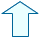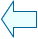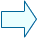The multiplication of fractions Fractions

1. The graphic of the multiplication of fractions.

If we represent a fraction on the horizontal axis and the other fraction on the vertical axis, the surface of the resulting rectangle will show us the value of the product of those fractions.

 Type the numerator and the denominator of a fraction above and the numerator and denominator of the other fraction below. Use small numbers so that it can be understood better.

- Count the resulting parts, this will be the numerator of the product.
- Count the parts forming a unit square, this will be the denominator of the product.
- What is the relation between the numerator of the product and the numerators of the factor fractions?
- What is the relation between the denominator of the product and the denominators of the factor fractions?
This way, you can deduce the method to obtain the product of two fractions numerically.Eduardo Barbero CorralSpanish Ministry of Education. Year 2007